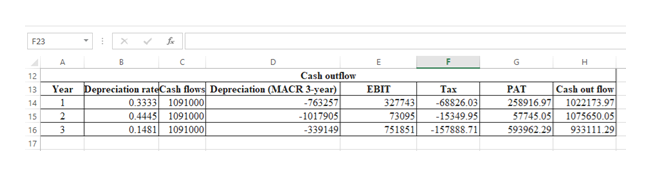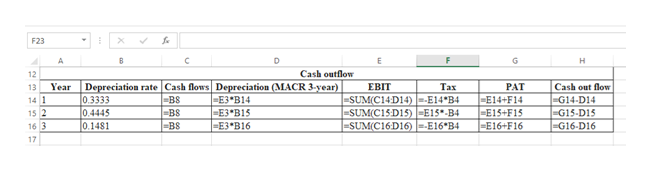# Down Under Boomerang, Inc., is considering a new 3-year expansion project that requires an initial fixed asset investment of \$2.29 million. The fixed asset falls into the 3-year MACRS class (MACRS schedule). The project is estimated to generate \$1,715,000 in annual sales, with costs of \$624,000. The project requires an initial investment in net working capital of \$260,000, and the fixed asset will have a market value of \$195,000 at the end of the project.  a.If the tax rate is 21 percent, what is the project’s Year 0 net cash flow? Year 1? Year 2? Year 3? (A negative answer should be indicated by a minus sign. Do not round intermediate calculations and enter your answers in dollars, not millions of dollars, rounded to two decimal places, e.g., 1,234,567.89.)b.If the required return is 9 percent, what is the project's NPV? (Do not round intermediate calculations and enter your answer in dollars, not millions of dollars, rounded to two decimal places, e.g., 1,234,567.89.)

Question
45 views
 Down Under Boomerang, Inc., is considering a new 3-year expansion project that requires an initial fixed asset investment of \$2.29 million. The fixed asset falls into the 3-year MACRS class (MACRS schedule). The project is estimated to generate \$1,715,000 in annual sales, with costs of \$624,000. The project requires an initial investment in net working capital of \$260,000, and the fixed asset will have a market value of \$195,000 at the end of the project.

 a. If the tax rate is 21 percent, what is the project’s Year 0 net cash flow? Year 1? Year 2? Year 3? (A negative answer should be indicated by a minus sign. Do not round intermediate calculations and enter your answers in dollars, not millions of dollars, rounded to two decimal places, e.g., 1,234,567.89.) b. If the required return is 9 percent, what is the project's NPV? (Do not round intermediate calculations and enter your answer in dollars, not millions of dollars, rounded to two decimal places, e.g., 1,234,567.89.)

check_circle

Step 1

a.)

Computation of net cash flows of year 0 to year 3:

Step 2

The cash outflow is as follows:help_outlineImage TranscriptioncloseF23 F A B C D E G H Cash outflow 12 Year Depreciation rateCash flows Depreciation (MACR 3-year) 0.3333 1091000 0.4445 1091000 0.1481 1091000 Cash out flow 1022173.97 1075650.05 ЕВT Тах PAT 13 -763257 -1017905 -339149 258916.97 57745.05 593962 29 327743 73095 751851 1 -68826.03 14 -15349.95 15 -157888.71 93311129 16 17 fullscreen
Step 3

Working note:

...help_outlineImage TranscriptioncloseF23 B C D F G A E н Cash outflow 12 Depreciation rate Cash flows Depreciation (MACR 3-year) 0.3333 0.4445 0.1481 Year ЕВIT Тах PAT E14+ F14 -E15+F15 -E16+ F16 Cash out flow G14-D14 |-G15-D15 G16-D16 13 |=E3*B14 -E3 B15 E3 B16 BS B8 =BS |=SUM(C14D14)--E14*B4 |-SUM(C15D15)E15-B4 -SUM(C16D16 E16 B4 14 1 15 2 16 3 17 fullscreen

### Want to see the full answer?

See Solution

#### Want to see this answer and more?

Solutions are written by subject experts who are available 24/7. Questions are typically answered within 1 hour.*

See Solution
*Response times may vary by subject and question.
Tagged in